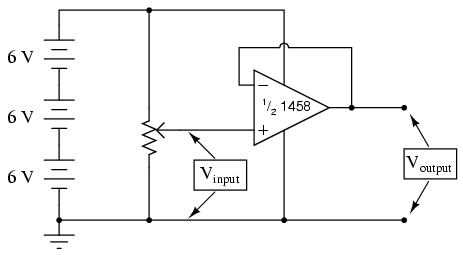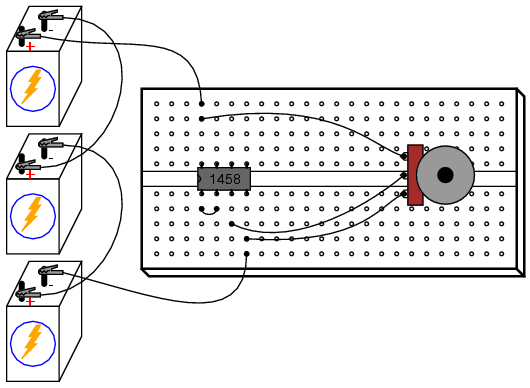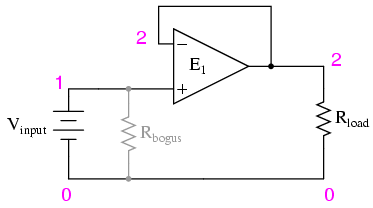» Experiment » Calculator/Converters » Radio » Newsletter » Associations and Societies » Component Manufacturers

 Electronics Symentics A B C D E F G H I J K L M N O P Q R S T U V W X Y Z

## Precision voltage follower

PARTS AND MATERIALS

• Operational amplifier, model 1458 or 353 recommended (Radio Shack catalog # 276-038 and 900-6298, respectively)

• Three 6 volt batteries

• One 10 kΩ potentiometer, linear taper (Radio Shack catalog # 271-1715)

CROSS-REFERENCES

Lessons In Electric Circuits, Volume 3, chapter 8: "Operational Amplifiers"

LEARNING OBJECTIVES

• How to use an op-amp as a voltage follower

• Purpose of negative feedback

• Troubleshooting strategy

SCHEMATIC DIAGRAMILLUSTRATIONINSTRUCTIONS

In the previous op-amp experiment, the amplifier was used in "open-loop" mode; that is, without any feedback from output to input. As such, the full voltage gain of the operational amplifier was available, resulting in the output voltage saturating for virtually any amount of differential voltage applied between the two input terminals. This is good if we desire comparator operation, but if we want the op-amp to behave as a true amplifier, we need it to exhibit a manageable voltage gain.

Since we do not have the luxury of disassembling the integrated circuitry of the op-amp and changing resistor values to give a lesser voltage gain, we are limited to external connections and componentry. Actually, this is not a disadvantage as one might think, because the combination of extremely high open-loop voltage gain coupled with feedback allows us to use the op-amp for a much wider variety of purposes, much easier than if we were to exercise the option of modifying its internal circuitry.

If we connect the output of an op-amp to its inverting (-) input, the output voltage will seek whatever level is necessary to balance the inverting input's voltage with that applied to the noninverting (+) input. If this feedback connection is direct, as in a straight piece of wire, the output voltage will precisely "follow" the noninverting input's voltage. Unlike the voltage follower circuit made from a single transistor (see chapter 5: Discrete Semiconductor Circuits), which approximated the input voltage to within several tenths of a volt, this voltage follower circuit will output a voltage accurate to within mere microvolts of the input voltage!

Measure the input voltage of this circuit with a voltmeter connected between the op-amp's noninverting (+) input terminal and circuit ground (the negative side of the power supply), and the output voltage between the op-amp's output terminal and circuit ground. Watch the op-amp's output voltage follow the input voltage as you adjust the potentiometer through its range.

You may directly measure the difference, or error, between output and input voltages by connecting the voltmeter between the op-amp's two input terminals. Throughout most of the potentiometer's range, this error voltage should be almost zero.

Try moving the potentiometer to one of its extreme positions, far clockwise or far counterclockwise. Measure error voltage, or compare output voltage against input voltage. Do you notice anything unusual? If you are using the model 1458 or model 353 op-amp for this experiment, you should measure a substantial error voltage, or difference between output and input. Many op-amps, the specified models included, cannot "swing" their output voltage exactly to full power supply ("rail") voltage levels. In this case, the "rail" voltages are +18 volts and 0 volts, respectively. Due to limitations in the 1458's internal circuitry, its output voltage is unable to exactly reach these high and low limits. You may find that it can only go within a volt or two of the power supply "rails." This is a very important limitation to understand when designing circuits using operational amplifiers. If full "rail-to-rail" output voltage swing is required in a circuit design, other op-amp models may be selected which offer this capability. The model 3130 is one such op-amp.

Precision voltage follower circuits are useful if the voltage signal to be amplified cannot tolerate "loading;" that is, if it has a high source impedance. Since a voltage follower by definition has a voltage gain of 1, its purpose has nothing to do with amplifying voltage, but rather with amplifying a signal's capacity to deliver current to a load.

Voltage follower circuits have another important use for circuit builders: they allow for simple linear testing of an op-amp. One of the troubleshooting techniques I recommend is to simplify and rebuild. Suppose that you are building a circuit using one or more op-amps to perform some advanced function. If one of those op-amps seems to be causing a problem and you suspect it may be faulty, try re-connecting it as a simple voltage follower and see if it functions in that capacity. An op-amp that fails to work as a voltage follower certainly won't work as anything more complex!

COMPUTER SIMULATION

Schematic with SPICE node numbers:Netlist (make a text file containing the following text, verbatim):

```Voltage follower
vinput 1 0
rbogus 1 0 1meg
e1 2 0 1 2 999meg
.dc vinput 5 5 1
.print dc v(1,0) v(2,0) v(1,2)
.end
```

An ideal operational amplifier may be simulated in SPICE using a dependent voltage source (e1 in the netlist). The output nodes are specified first (2 0), then the two input nodes, non-inverting input first (1 2). Open-loop gain is specified last (999meg) in the dependent voltage source line.

Because SPICE views the input impedance of a dependent source as infinite, some finite amount of resistance must be included to avoid an analysis error. This is the purpose of Rbogus: to provide DC path to ground for the Vinput voltage source. Such "bogus" resistances should be arbitrarily large. In this simulation I chose 1 MΩ for an Rbogus value.

A load resistor is included in the circuit for much the same reason: to provide a DC path for current at the output of the dependent voltage source. As you can see, SPICE doesn't like open circuits!